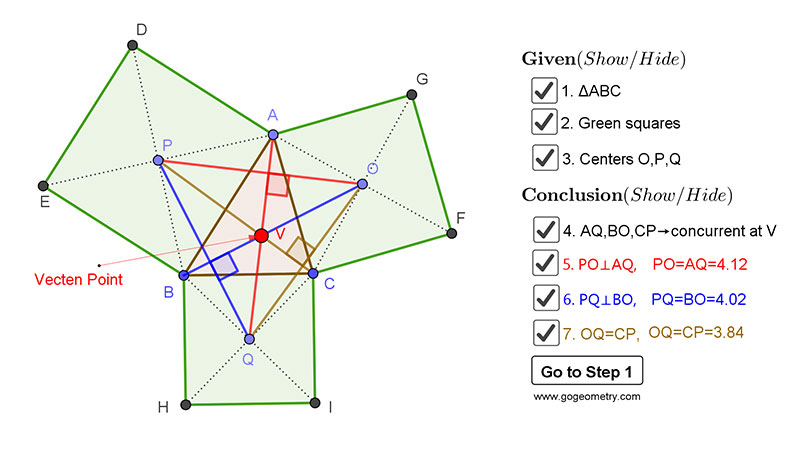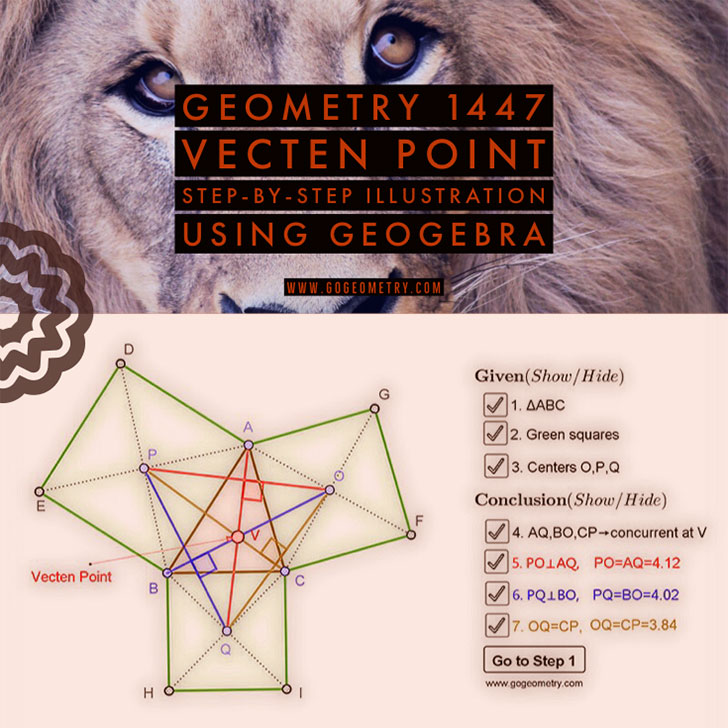# Dynamic Geometry Problem 1447: Outer Vecten Point

Given a triangle ABC with three squares erected externally on the sides AB, AC, and BC with centers P, O, and Q, respectively. Then (1) the lines AQ, BO, and CP are concurrent at a point V, called the outer Vecten point of the triangle ABC. (2) AQ is equal and perpendicular to PO, BO is equal and perpendicular to PQ, CP is equal and perpendicular to OQ.

In 1817, M. Vecten, was a French Mathematician, who taught mathematics with Gergonne in Nımes,France.
The outer Vecten point is the point X(485) in Clark Kimberling's Encyclopedia of Triangle Centers.

## Static Diagram of the outer Vecten point of a triangle## Poster of the Outer Vecten Point using iPad Apps### Classroom Resource:Interactive step-by-step animation using GeoGebra

This step-by-step interactive illustration was created with GeoGebra.

• To explore (show / hide): click/tap a check box.
• To stop/play the animation: click/tap the icon in the lower left corner.
• To go to first step: click/tap the "Go to step 1" button.
• To manipulate the interactive figure: click/tap and drag the blue points or figures.

GeoGebra is free and multi-platform dynamic mathematics software for all levels of education that joins geometry, algebra, tables, graphing, statistics and calculus application, intended for teachers and students. Many parts of GeoGebra have been ported to HTML5.